Back to Kemp Acoustics HomeNext: Radiation impedance Up: Multimodal propagation in acoustic Previous: Projection along a cylinder   Contents

# Method for calculation of pressure field

If we set
and
for
at the mouth of the horn, we have a plane velocity at the input end of the horn. Physically this corresponds to driving the input with a rigid piston. Using stored values of the impedance along the guide we can project the volume velocity vector forward to the end of the guide using the following equations:
(2.102)

whereis a diagonal matrix with theth diagonal given by
and
is a diagonal matrix with theth diagonal given by.
(2.103)

The pressure vector at each point along the horn is then given by
.

The entries in the vector give the complex amplitude of each mode. Consider the time dependence of the plane wave component of the pressure at the input, having a complex amplitude
:
(2.104)

This will have a maximum amplitude of
and vary sinusoidally in time:
(2.105)

where
is the angle of the pressure on the complex plane at:(2.106)

Since the plane wave term in the volume velocity vector was chosen to be real at the input end, the volume velocity is at its maximum atand the phase anglefor the plane component of the pressure vector at the input gives the phase angle by which the pressure leads the volume velocity. We will choose to plot the pressure field when the pressure at the input is at its maximum. From equation (2.105) we see that this occurs at time
.

Now consider the pressure at some point along the length of the duct where the complex pressure amplitude of theth mode is:
(2.107)

Puttingand taking the real part gives the physically observable pressure field when the plane pressure is maximum at the input:(2.108)

Figure 2.9 shows the pressure field calculated in this manner for a cylinder of length 5mm and radius
mm driven by a piston vibrating sinusoidally at 10 kHz connected to a cylinder of radius
mm assuming lossy propagation. 25 modes were used and the system was approximated by 1000 cylinders for the calculation. The termination on the right is the infinite cylindrical pipe termination
where. Here red indicates the maximum value of the real part of the pressure and blue the minimum.

Notice that the wavefronts expand out from the opening. The contours of equal pressure are perpendicular to the walls as required by the hard walled boundary condition. The pressure is continuous at the discontinuity showing that the algorithm correctly projects the modes across.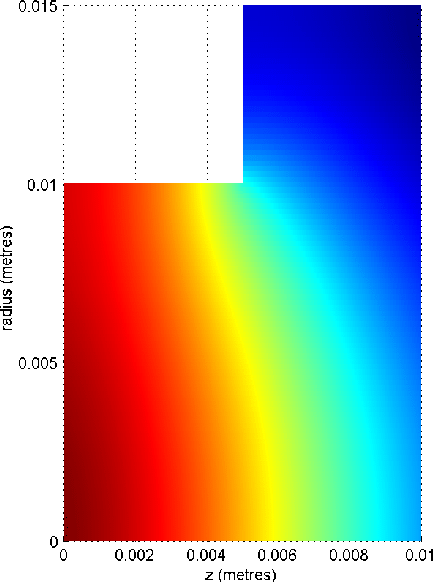Back to Kemp Acoustics HomeNext: Radiation impedance Up: Multimodal propagation in acoustic Previous: Projection along a cylinder   Contents
Jonathan Kemp 2003-03-24